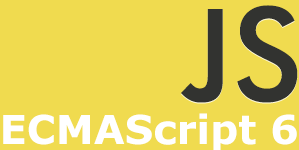##Arrow functions provide a terse syntax, making for code that is more concise and flexible.

One of the most significant features of the ECMAScript 6 specification is arrow functions. Also referred to sometimes as “fat functions,” this change radically alters the rules with regard to functions in JavaScript. Initially, I had planned on writing an “Introduction to / Overview” article about arrow functions. But since code samples are always helpful, I didn’t want an “Overview” article that had code examples for every aspect of arrow functions. Instead, I decided to just jump in, and create an article that focuses on each area of importance. So this article provides a very simple introduction to general arrow function syntax.

Perhaps the hardest part to get used to with regard to ECMAScript 6 arrow function syntax is the fact that the “function” keyword and parameters are reversed. In addition, the “function” keyword is replaced by: “=>”. So, the end result is:

The above example is pseudo code, but my intention was to demonstrate the correct syntax for arrow functions. So let’s look at a working example:

## Example # 1B

In Example # 1A, we have the standard syntax you would use when creating an anonymous function expression. That is, the function “addOne” takes a single parameter (a number), and returns that number plus one.

Now in Example # 1B, we have accomplished the exact same result, using arrow function syntax. The “var addOne =” portion of the code should be familiar to you. But it’s after the assignment operator, that things start to look quite different. First, we next have the single parameter: “someNumber”. That parameter is followed by: “=>”. This equal+greater than character combination replaces the “function” keyword. Next we have the function body.

## The Function Body

In Example # 1B, you may have noticed the absence of the curly braces. This is because the body of our arrow function consisted of one expression. In this case, the curly braces can be omitted. When the arrow function consists of more than one expression, then curly braces are required. Also, when the function body consists of a single expression, the implicit return value of the function is the result of that expression. This is why the return value of our arrow function is the passed-in parameter plus one, even though there is no “return” statement.

## Example # 2 B

In Example # 2A, the return value of addOne(2) is: “undefined”. This happens because the function body of addOne has curly braces, but we have not explicitly returned a value. So, just as with any function in JavaScript, a function that does not specify a return value returns “undefined” (the exception to this rule is a constructor function, which can return any object but returns the instance object when no return value is specified).

In Example # 2B, we get the expected behaviour because we specify a return value.

## Returning an Object in a Single-Expression Function Body

One of the main benefits of arrow function is the terse syntax. While multi-expression function bodies are perfectly acceptable, it is likely that in many cases, you’ll want to leverage that streamline single-expression (hence single-line) syntax. But what if you want to return an object in this scenario? Since the curly braces can be omitted, you simply need to wrap the object you return in parentheses.

## Example # 3

In Example # 3, we’ve gone back to a single-line version of the addOne arrow function. What differs here is that the function body is wrapped in a set of parentheses. Inside of these parentheses is an object. The beauty of this approach is that the single-line version of arrow functions allows you to omit the “return” statement, so we just provide the object that we want to return in the parentheses.

### Summary

This article merely scratches the surface of what is possible in ECMAScript 6 Arrow functions. But the goal here was to simply provide a gentle introduction to the syntax. The biggest changes you’ll notice right away are:

• The order of the parameter(s) and the “function” keyword are switched.
• The “function” keyword has been replaced by: “=>”.
• When the function body has a single expression, no curly braces are required.
• When the function body has a single expression, the implied return value is the expression.
• When the function body has a single expression, an object can be implicitly returned if it is wrapped in parentheses.

In my next post, I’ll dive a bit deeper into Arrow Function parameters.